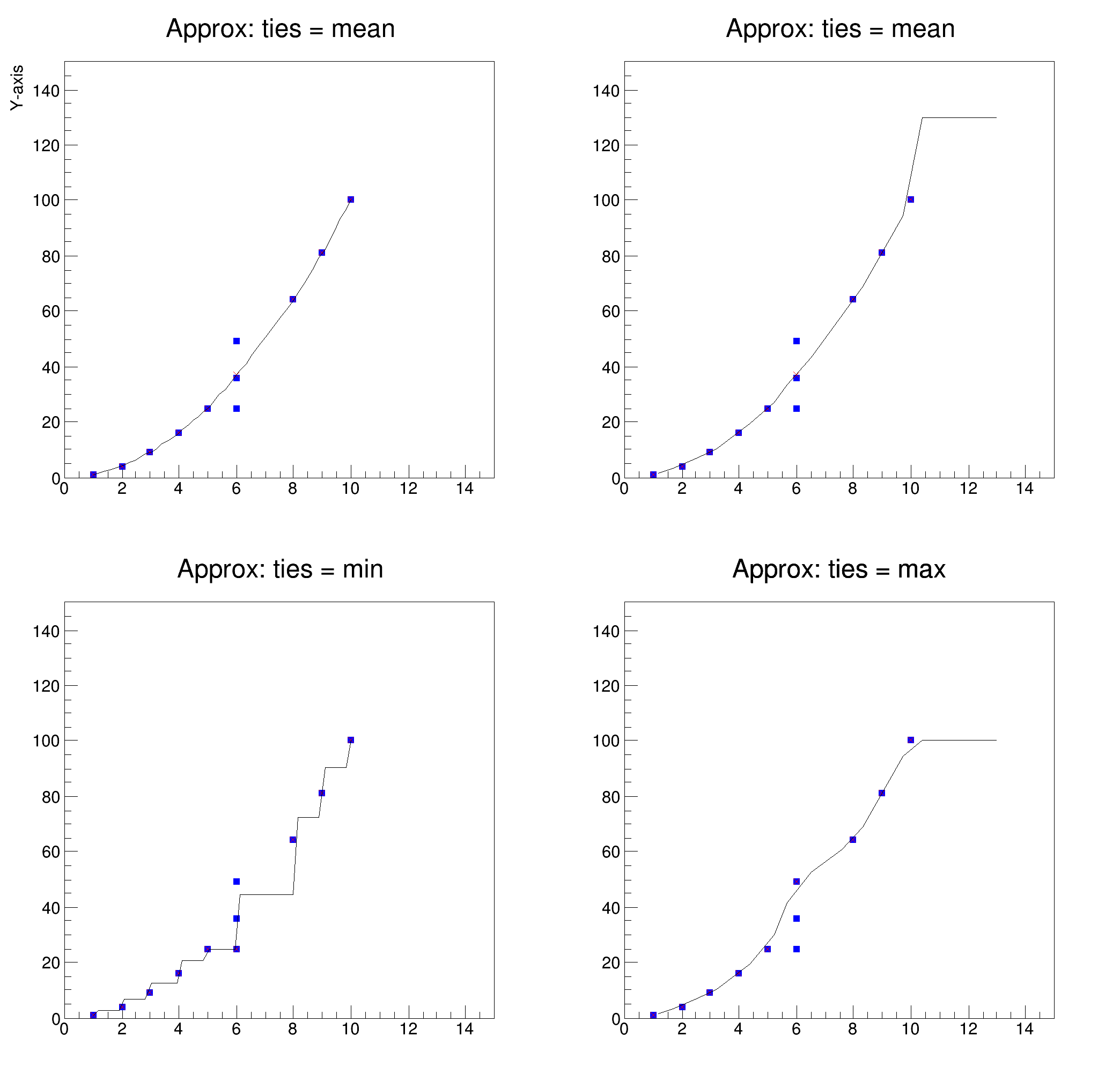ROOT   Reference Guideapprox.C File Reference

## Detailed DescriptionMacro to test interpolation function ApproxTCanvas *vC1;
TGraph *grxy, *grin, *grout;
void DrawSmooth(Int_t pad, const char *title, const char *xt, const char *yt)
{
vFrame->SetTitle(title);
vFrame->SetTitleSize(0.2);
vFrame->SetXTitle(xt);
vFrame->SetYTitle(yt);
grxy->SetMarkerStyle(21);
grxy->SetMarkerSize(0.5);
grxy->Draw("P");
grin->SetMarkerStyle(5);
grin->SetMarkerSize(0.7);
grin->Draw("P");
grout->DrawClone("LP");
}
void approx()
{
// Test data (square)
Int_t n = 11;
Double_t x[] = {1,2,3,4,5,6,6,6,8,9,10};
Double_t y[] = {1,4,9,16,25,25,36,49,64,81,100};
grxy = new TGraph(n,x,y);
// X values, for which y values should be interpolated
Int_t nout = 14;
Double_t xout[] =
{1.2,1.7,2.5,3.2,4.4,5.2,5.7,6.5,7.6,8.3,9.7,10.4,11.3,13};
// Create Canvas
vC1 = new TCanvas("vC1","square",200,10,700,700);
vC1->Divide(2,2);
// Initialize graph with data
grin = new TGraph(n,x,y);
// Interpolate at equidistant points (use mean for tied x-values)
TGraphSmooth *gs = new TGraphSmooth("normal");
grout = gs->Approx(grin,"linear");
DrawSmooth(1,"Approx: ties = mean","X-axis","Y-axis");
// Re-initialize graph with data
// (since graph points were set to unique vales)
grin = new TGraph(n,x,y);
// Interpolate at given points xout
grout = gs->Approx(grin,"linear", 14, xout, 0, 130);
DrawSmooth(2,"Approx: ties = mean","","");
// Print output variables for given values xout
Int_t vNout = grout->GetN();
Double_t vXout, vYout;
for (Int_t k=0;k<vNout;k++) {
grout->GetPoint(k, vXout, vYout);
cout << "k= " << k << " vXout[k]= " << vXout
<< " vYout[k]= " << vYout << endl;
}
// Re-initialize graph with data
grin = new TGraph(n,x,y);
// Interpolate at equidistant points (use min for tied x-values)
// _grout = gs->Approx(grin,"linear", 50, 0, 0, 0, 1, 0, "min");_
grout = gs->Approx(grin,"constant", 50, 0, 0, 0, 1, 0.5, "min");
DrawSmooth(3,"Approx: ties = min","","");
// Re-initialize graph with data
grin = new TGraph(n,x,y);
// Interpolate at equidistant points (use max for tied x-values)
grout = gs->Approx(grin,"linear", 14, xout, 0, 0, 2, 0, "max");
DrawSmooth(4,"Approx: ties = max","","");
// Cleanup
delete gs;
}
int Int_t
Definition: RtypesCore.h:45
double Double_t
Definition: RtypesCore.h:59
@ kRed
Definition: Rtypes.h:66
@ kBlue
Definition: Rtypes.h:66
virtual void SetMarkerColor(Color_t mcolor=1)
Set the marker color.
Definition: TAttMarker.h:38
virtual void SetMarkerStyle(Style_t mstyle=1)
Set the marker style.
Definition: TAttMarker.h:40
virtual void SetMarkerSize(Size_t msize=1)
Set the marker size.
Definition: TAttMarker.h:41
The Canvas class.
Definition: TCanvas.h:23
Definition: TCanvas.cxx:708
A helper class to smooth TGraph.
Definition: TGraphSmooth.h:36
TGraph * Approx(TGraph *grin, Option_t *option="linear", Int_t nout=50, Double_t *xout=0, Double_t yleft=0, Double_t yright=0, Int_t rule=0, Double_t f=0, Option_t *ties="mean")
Approximate data points.
A TGraph is an object made of two arrays X and Y with npoints each.
Definition: TGraph.h:41
Int_t GetN() const
Definition: TGraph.h:124
virtual void Draw(Option_t *chopt="")
Draw this graph with its current attributes.
Definition: TGraph.cxx:769
virtual Int_t GetPoint(Int_t i, Double_t &x, Double_t &y) const
Get x and y values for point number i.
Definition: TGraph.cxx:1607
1-D histogram with a float per channel (see TH1 documentation)}
Definition: TH1.h:575
virtual void SetTitle(const char *title)
Definition: TH1.cxx:6678
virtual void SetTitleSize(Float_t size=0.02, Option_t *axis="X")
Set the axis' title size.
Definition: Haxis.cxx:365
virtual void SetXTitle(const char *title)
Definition: TH1.h:413
virtual void SetYTitle(const char *title)
Definition: TH1.h:414
virtual TObject * DrawClone(Option_t *option="") const